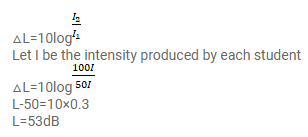# The noise level in a classroom in absence of the teacherQuestion:

The noise level in a classroom in absence of the teacher is $50 \mathrm{~dB}$ when 50 students are present. Assuming that on the average each student output same sound energy per second, what will be the noise level if the number of students is increased to 100 ?

Solution: